# Cluster Sampling in Pandas

• Last Updated : 01 Apr, 2021

Sampling is a method in which we collect or chosen a small set of data from a large population, without finding the meaning of every individual in set. We’re doing a sampling of data from the population because we cannot gather data from the entire population. If we’re done sampling then we bring the population into manageable form and to help in minimizing error due to the large number in the population.

We can do sampling with several methods, here we only discuss cluster sampling:

### Cluster sampling:

Cluster sampling is a type of probability sampling in which every and each element of the population is selected equally, we use the subsets of the population as the sampling part rather than the individual elements for sampling.

The population is divided into subsets or subgroups that are considered as clusters, and from the numbers of clusters, we select the individual cluster for the next step to be performed.

Now see the steps taken to perform cluster sampling on the population:

Step 1: Define and identify the target population.

This is the first step we have to perform for sampling in this we have to clearly select the targeted area from the population.

For understanding in easy language let’s take an example of integers, integers are the number which can be written without fractional form like all negative and positive numbers ( -1,-4,-8,3,5,2,0……etc ) so we take all the integers as our data and from this data we have to select our targeted area for performing the sampling. From these data of integers, we can target either all the positive numbers or all the negative.

Here we assume that our targeted area is all positive numbers means we take all positive numbers from integers data as our sample.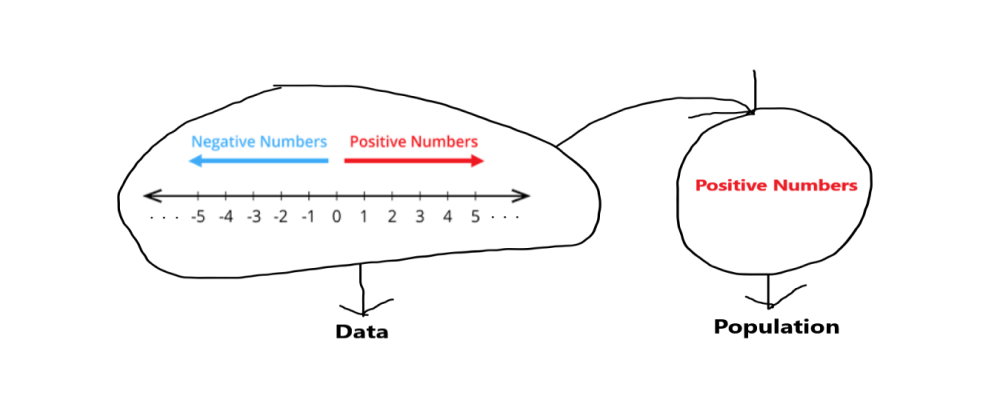Step 2: Sampling method.

Here we use probability cluster sampling because every element from the population has an equal chance to select.

Step 3: Divide samples into clusters.

After we select the sampling method we divide samples into clusters, it is an important part of performing cluster sampling we have to create a quality cluster as they produce better accuracy after sampling. we remind that clusters we are producing have the better impact it means they represent well to our population. Clusters have to be equally and similarly distributed as there is no repetition among them. Ideally, each cluster should be a mini-representation of the entire population.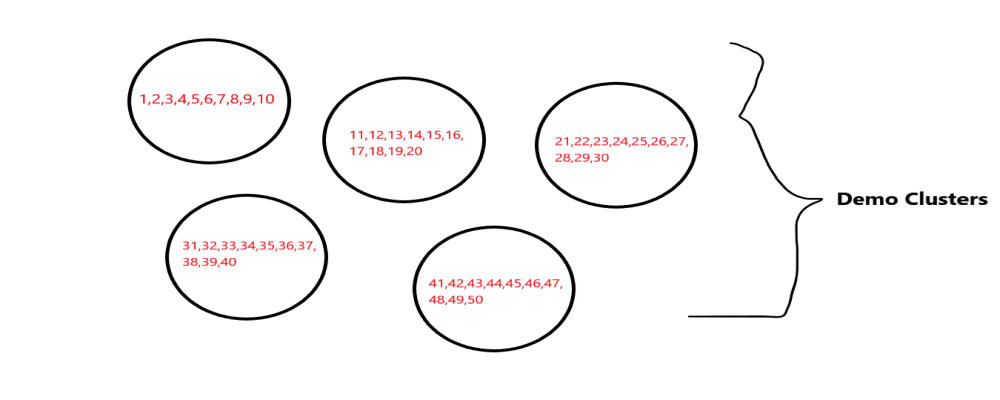Step 4: Collect data.

In the last step after performing the above steps, we collect our desirable data from the sample.

We take an example of cluster sampling in which we take 1 to n natural numbers that will make clusters and from that cluster, we select the random individual clusters for sampling.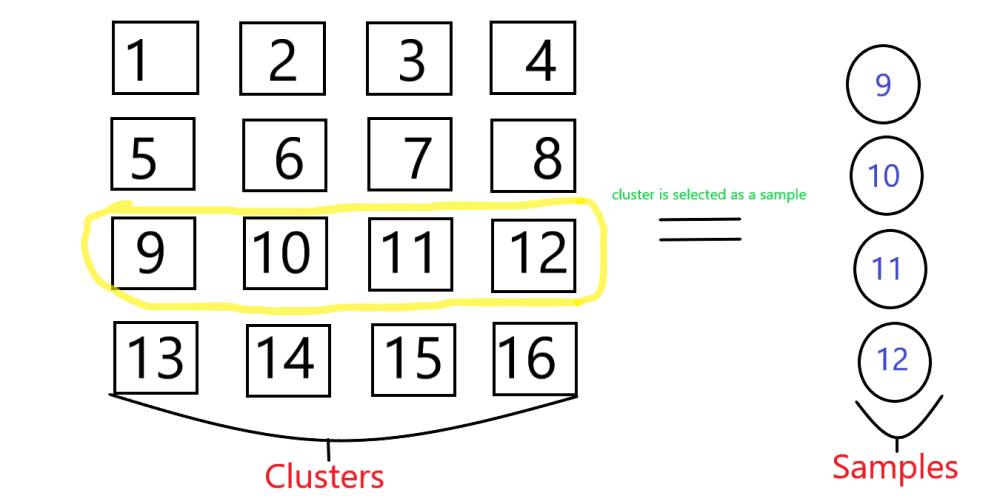N=16, so we take samples from 1 to 16 and having an individual cluster of 4 numbers after that random from the clusters we select one cluster as a sample.

Example 1:

## Python3

 `# import pandas``import` `pandas as pd`` ` `# import numpy``import` `numpy as np`` ` `# creating dictionary of data``data ``=` `{``'N_numbers'``:np.arange(``1``,``16``)}`` ` `# creating dataframe``df ``=` `pd.DataFrame(data)`` ` `# sample of data``samples ``=` `df.sample(``4``)``print``(samples)`

Output: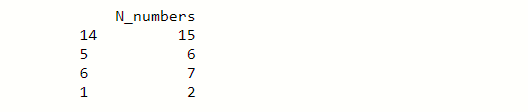Random samples of 4 numbers form the cluster of 1-16 numbers.

In this output, we see that from the N-numbers column having random numbers, by the way, this is our random cluster which contains 4 samples. With the help of Sample() set the no of samples that an individual cluster presents.

Example 2:

## Python3

 `# code``# importing modules``import` `pandas as pd``import` `numpy as np`` ` `#creating dictionary of data``dic_data ``=` `{``'employee_id'``:np.arange(``1``,``21``),``                  ``'value'``:np.random.randn(``20``)}`` ` `# creating DataFrame from dictonary``df ``=` `pd.DataFrame(dic_data)``print``(df)`

Output: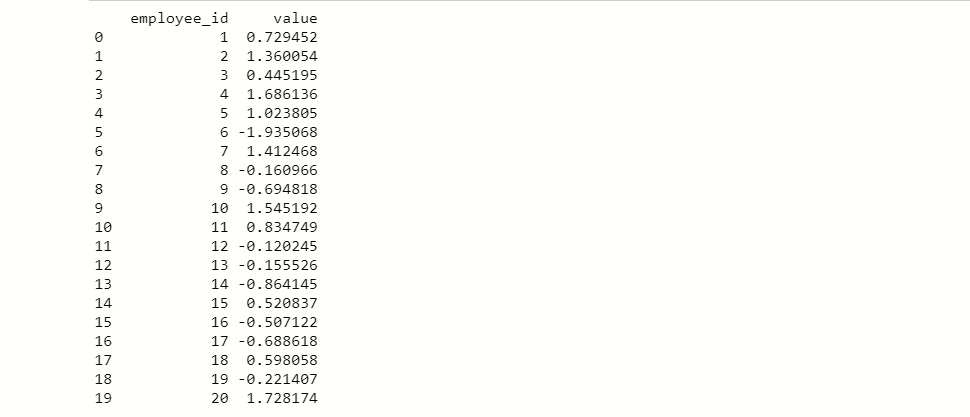Here we create DataFrame df which contain 20 employee id and each id having random values. From the dataset df, we take a random cluster that contains specific 4 random samples.

Code:

## Python3

 `# code``# import pandas ``import` `pandas as pd`` ` `# import numpy ``import` `numpy as np`` ` `# creating samples``samples ``=` `df.sample(``4``).sort_values(by``=``'employee_id'``)`` ` `# show samples``print``(samples)`

Output: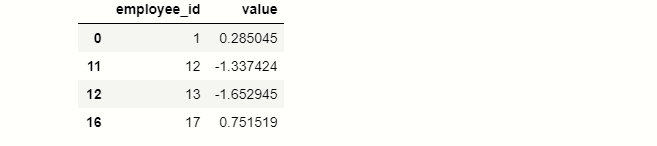These are the randomly selected employee

As you can see in our output that we collect data of 4 random employees which contain their employee_id and value. First, we create a sample variable and then assign it a sample of 4 random employees which acts as our data.

My Personal Notes arrow_drop_up